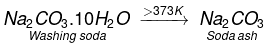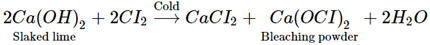Courses

# NCERT Exemplar - s-block elements NEET Notes | EduRev

## JEE : NCERT Exemplar - s-block elements NEET Notes | EduRev

The document NCERT Exemplar - s-block elements NEET Notes | EduRev is a part of the JEE Course JEE Revision Notes.
All you need of JEE at this link: JEE

Multiple Choice Questions-II

Q.1. The alkali metals are low melting. Which of the following alkali metal is expected to melt if the room temperature rises to 30°C?
(a) Na
(b) K
(c) Rb
(d) Cs
Ans. (d)
Solution:
Among alkali metals, the melting point decreases as the strength of metallic bonding decreases with increasing size of the atom. Thus, Cs has the lowest melting point (28.5°C) and will melt at 30°C.

Q.2. Alkali metals react with water vigorously to form hydroxides and dihydrogen. Which of the following alkali metals reacts with water least vigorously?
(a) Li
(b) Na
(c) K
(d) Cs
Ans.
(a)
Solution:
Both melting point and heat of reaction of alkali metals with water decrease down the group from Li to Cs. Although the heat of reaction of Li is the highest, but due to its high melting point, even this heat is not sufficient to melt the metal, which exposes greater surface to water for reaction.

As a result, Li has the least reactivity but the reactivity increases as the melting point of alkali metals decreases down the group from Li to Cs.

Q.3. The reducing power of a metal depends on various factors. Suggest the factor which makes Li, the strongest reducing agent in aqueous solution.
(a) Sublimation enthalpy
(b) Ionisation enthalpy
(c) Hydration enthalpy
(d) Electron-gain enthalpy
Ans.
(c)
Solution: Lithium has highest hydration enthalpy which accounts for its high negative E° value and its high reducing power.

Q.4. Metal carbonates decompose on heating to give metal oxide and carbon dioxide. Which of the metal carbonates is most stable thermally?
(a) MgCO3
(b) CaCO3
(c) SrCO3
(d) BaCO3
Ans.
(d)
Solution:
Thermal stability of metal carbonates increases as the electropositive character of the metal or the basicity of the metal hydroxide increases from Be(OH)2 to Ba(OH)2. Thus, BaCO3 is the most stable.

Q.5. Which of the carbonates given below is unstable in air and is kept in CO2 atmosphere to avoid decomposition.
(a) BeCO3
(b) MgCO3
(c) CaCO3
(d) BaCO3
Ans.
(a)
Solution:
Due to least electropositive character or least basicity of Be, BeCO3 is less stable and hence decomposes to give BeO and CO2.
BeCO→ BeO + CO2
Since the decomposition reaction is reversible, therefore, to increase the stability of BeCO3 or to reverse the above equilibrium, BeCO3 is kept in an atmosphere of CO2.

Q.6. Metals form basic hydroxides. Which of the following metal hydroxide is the least basic?
(a) Mg(OH)2
(b) Ca(OH)2
(c) Sr(OH)2
(d) Ba(OH)2

Ans. (a)
Solution: As the ionization enthalpy increases from Mg →Ba, the M – O bond becomes weaker and weaker down the group and hence basicity increases down the group. Thus, Mg(OH)2 is least basic.

Q.7. Some of the Group 2 metal halides are covalent and soluble in organic solvents. Among the following metal halides, the one which is soluble in ethanol is
(a) BeCl2
(b) MgCl2
(c) CaCl2
(d) SrCl2

Ans. (a)
Solution: Due to small size, high electronegativity and high ionization enthalpy of Be, BeCl2 is covalent and hence most soluble in organic solvents such as ethanol.

Q.8.The order of decreasing ionisation enthalpy in alkali metals is
(a) Na > Li > K > Rb
(b) Rb <  Na < K < Li
(c) Li > Na > K > Rb
(d) K < Li < Na < Rb
Ans.
(c)
Solution:
Ionization enthalpy decreases with increase in Size of the atom in a group. Hence, the order is: Li > Na > K > Rb.

Q.9. The solubility of metal halides depends on their nature, lattice enthalpy and hydration enthalpy of the individual ions. Amongst fluorides of alkali metals, the lowest solubility of LiF in water is due to
(a) Ionic nature of lithium fluoride
(b) High lattice enthalpy
(c) High hydration enthalpy for lithium-ion.
(d) Low ionisation enthalpy of lithium atom
Ans.
(b)
Solution:
Due to small size of Li+ and F ions, lattice enthalpy is much higher than hydration enthalpy and hence LiF is least soluble among alkali metal fluorides.

Q.10. Amphoteric hydroxides react with both alkalies and acids. Which of the following Group 2 metal hydroxides is soluble in sodium hydroxide?
(a) Be(OH)2
(b) Mg(OH)2
(c) Ca(OH)2
(d) Ba(OH)2
Ans.
(a)
Solution: Be(OH)2 reacts with NaOH to give beryllate ion, becoming soluble in it.
Be(OH)2 + 2OH- → [Be(OH)4]2

Q.11. In the synthesis of sodium carbonate, the recovery of ammonia is done by treating NH4Cl with Ca(OH)2. The by-product obtained in this process is
(a) CaCl2
(b) NaCl
(c) NaOH
(d) NaHCO3
Ans.
(a)
Solution:
In solvay ammonia soda process, Na2COis manufactured. The reactions involved in the synthesis are:
NH3 + H2O + CO2 → NH4HCO3
NH4HCO3 + NaCl → NaHCO3 + NH4Cl
2NaHCO→ Na2CO+ H2O
NHis recovered from NH4HCOand NH4Cl and in this process CaClis formed as a by product according to the following reactions:
NH4HCO3(on heating) → NH+ H2O + CO2
2NH4Cl + Ca(OH)→ 2NH+ 2H2O + CaCl2

Q.12. When sodium is dissolved in liquid ammonia, a solution of deep blue colour is obtained. The colour of the solution is due to
(a) Ammoniated electron
(b) Sodium ion
(c) Sodium amide
(d) Ammoniated sodium ion

Ans. (a)
Solution: M+(x+y)NH→ M+(NH3)x + e(NH3)y
The colour of solution (deep blue) is due to the ammoniated electron which absorbs energy in the visible region.

Q.13. By adding gypsum to cement
(a) Setting time of cement becomes less.
(b) Setting time of cement increases.
(c) Colour of cement becomes light.
(d) Shining surface is obtained.
Ans.
(b)
Solution:
Raw materials for cement are limestone, clay and gypsum. Cement is a dirty greyish heavy powder containing calcium aluminates and silicates. Gypsum (CaSO4 - 2H2O) is added to the components to increase the setting time of cement so that it gets sufficiently hardened. The setting of cement is an exothermic process and involves hydration of calcium aluminates and, silicates.

Q.14. Dead burnt plaster is
(a) CaSO4
(b) CaSO4. 1/2 H2O
(c) CaSO4.H2O
(d) CaSO4.2H2O
Ans.
(a)
Solution: Plaster of Paris (CaSO4.1/2 H2O) on heating at 200°C changes into anhydrous CaSO4.

Q.15. Suspension of slaked lime in water is known as
(a) Lime water
(b) Quick lime
(c) Milk of lime
(d) Aqueous solution of slaked lime
Ans.
(c)
Solution: Suspension of slaked lime in water is known as milk of lime.

The reaction is CaO + H2O → Ca(OH)2

Q.16. Which of the following elements does not form hydride by direct heating with dihydrogen?
(a) Be
(b) Mg
(c) Sr
(d) Ba
Ans.
(a)
Solution: Due to high ionization enthalpy and small size, Be does not react with hydrogen by direct heating.

Q.17. The formula of soda ash is
(a) Na2CO3.10H2O
(b) Na2CO3.2H2O
(c) Na2CO3.H2O
(d) Na2CO3
Ans.
(d)
Solution:
On heating washing soda, it loses its water of crystallization. Above 373 K, it becomes completely anhydrous white powder called soda ash.Q.18. A substance which gives brick red flame and breaks down on heating to give oxygen and a brown gas is
(a) Magnesium nitrate
(b) Calcium nitrate
(c) Barium nitrate
(d) Strontium nitrate

Ans. (b)
Solution: 2Ca(NO3)→ 2CaO + 4NO2 + O2
NO2 is a brown gas. Ca2+ imparts brick red colour to the flame.

Q.19. Which of the following statements is true about Ca(OH)2?
(a) It is used in the preparation of bleaching powder
(b) It is a light blue solid
(c) It does not possess disinfectant property.
(d) It is used in the manufacture of cement.
Ans.
(a)
Solution:
Calcium hydroxide is used in the manufacture of bleaching powder.Q.20. A chemical A is used for the preparation of washing soda to recover ammonia. When CO2 is bubbled through an aqueous solution of A, the solution turns milky. It is used in whitewashing due to disinfectant nature. What is the chemical formula of A?
(a) Ca (HCO3)2
(b) CaO
(c) Ca(OH)2
(d) CaCO3
Ans.
(c)
Solution:
Chemical A used in the preparation of washing soda Na2CO3 to recover ammonia is Ca(OH)2. It is a reaction involved in the Solvay process for preparation of sodium carbonate.
2NH4Cl + Ca(OH)2 2NH3 + CaCl2 + H2O
When CO2 is bubbled through a solution of Ca(OH)2, it forms milky precipitate of CaCO3.
Ca(OH)2 + CO2 CaCO3 + H2O
Ca(OH)2 is used as slaked lime as white wash due to its disinfectant nature.

Q.21. Dehydration of hydrates of halides of calcium, barium and strontium i.e., CaCl26H2O, BaCl2.2H2O, SrCl2.2H2O, can be achieved by heating. These become wet on keeping in air. Which of the following statements is correct about these halides?
(a) Act as dehydrating agent
(b) Can absorb moisture from air
(c) Tendency to form hydrate decreases from calcium to barium
(d) All of the above
Ans.
(d)
Solution:
Chlorides of alkaline earth metals are hydrated salts. Due to their hygroscopic nature, they can be used as dehydrating agents to absorb moisture from air.
Extent of hydration decreases from Mg to Ba, i.e., MgCl2.6H2O, CaCl2.6H2O, BaCl2 2H2O SrCl2.2H2O.

Multiple Choice Questions - II

In the following questions, two or more options may be correct.
Q.22. Metallic elements are described by their standard electrode potential, fusion enthalpy, atomic size, etc. The alkali metals are characterised by which of the following properties?

(a) High boiling point
(b) High negative standard electrode potential
(c) High density
(d) Large atomic size
Ans.
(b, d)
Solution:
Alkali metals have high negative standard electrode potential and large atomic size.

Q.23. Several sodium compounds find use in industries. Which of the following compounds are used for textile industry?
(a) Na2CO3
(b) NaHCO3
(c) NaOH
(d) NaCl

Ans. (a, c)
Solution: Na2CO3 and NaOH are used in the textile industry.

Q.24. Which of the following compounds are readily soluble in water?
(a) BeSO4
(b) MgSO4
(c) BaSO4
(d) SrSO4
Ans.
(a, b)
Solution:
Solubility decreases down the group because hydration enthalpy decreases more rapidly than lattice enthalpy. Thus, BeSO4 and MgSO4 are soluble.

Q.25. When Zeolite, which is hydrated sodium aluminium silicate is treated with hard water, the sodium ions are exchanged with which of the following ion(s)?
(a) H+ ions
(b) Mg2+ ions
(c) Ca2+ ions
(d) SO2-ions

Ans.(b, c)
Solution: Sodium zeolite removes Ca2+ and Mg2+ ions from hard water.

Na2Z + M2+ → MZ + 2Na+(M = Ca, Mg) where, Z = Al2Si2O8H2O

Q.26. Identify the correct formula of halides of alkaline earth metals from the following.
(a) BaCl2.2H2O
(b) BaCl2.4H2O
(c) CaCl2.6H2O
(d) SrCl2.4H2O
Ans.
(a, c)
Solution:
Tendency to form halide hydrates gradually decreases down the group. The hydrates are MgCl2.6H2O, CaCl2.6H2O, SrCl2.6H2O and BaCl2.2H2O.

Q.27. Choose the correct statements from the following.
(a) Beryllium is not readily attacked by acids because of the presence of an oxide film on the surface of the metal.
(b) Beryllium sulphate is readily soluble in water as the greater hydration enthalpy of Be2+ overcomes the lattice enthalpy factor.
(c) Beryllium exhibits coordination number more than four.
(d) Beryllium oxide is purely acidic in nature.

Ans. (a, b)
Solution: Be does not exhibit coordination number more than four and BeO is amphoteric in nature.

Q.28. Which of the following are the correct reasons for the anomalous behaviour of lithium?
(a) Exceptionally small size of its atom
(b) Its high polarising power
(c) It has high degree of hydration
(d) Exceptionally low ionisation enthalpy
Ans.
(a, b)
Solution: Anomalous behaviour of Li is due to its exceptionally small size and high polarizing power.

Offer running on EduRev: Apply code STAYHOME200 to get INR 200 off on our premium plan EduRev Infinity!

233 docs

,

,

,

,

,

,

,

,

,

,

,

,

,

,

,

,

,

,

,

,

,

;